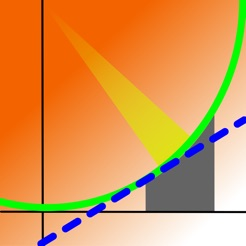## Screenshots

•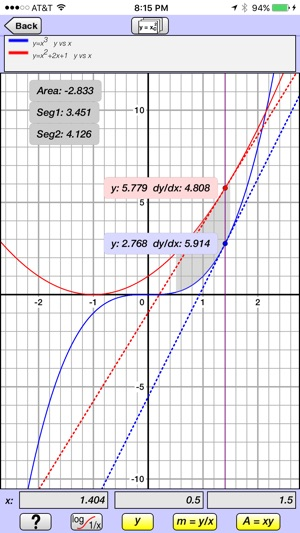•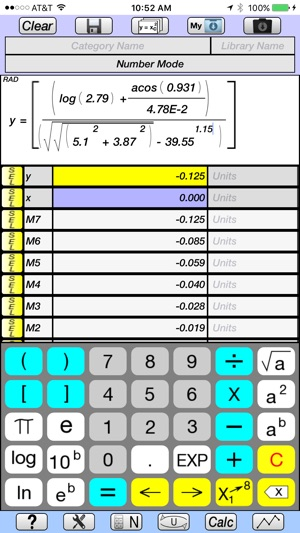••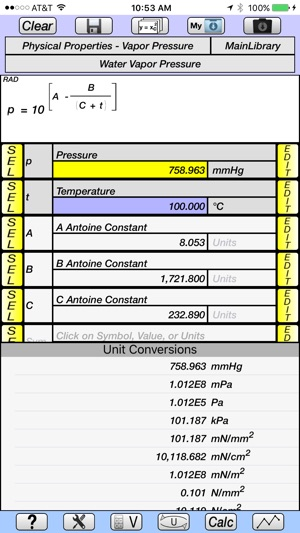•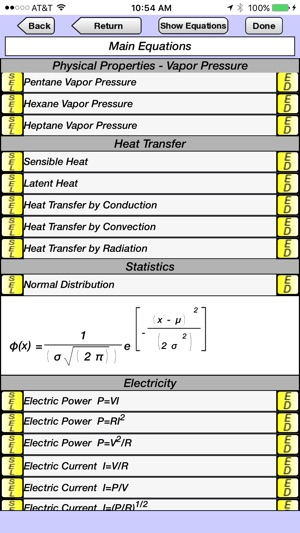## Description

V-Graph Save is a scientific graphing calculator for iPhone and iPad that is designed to solve real problems in engineering and science.

(V-Solve is a free app that has all of the features of V-Graph Save except for graphing and being able to save equations to the library. V-Solve has a demo feature which shows the graphing features of V-Graph Save for a select set of equations.)

Overview of Features

• Solves and plots explicit and implicit equations.
• Equations are entered and displayed in their natural form, as they would appear in a textbook.
• 59 categories of units in library, divided into english, metric, and SI systems.
• Equations, constants, and user-defined unit conversions can be saved into libraries.
• Well-organized Main, Trigonometric, English, and Greek keypads.
• Help screen overlay provides pop-up description of the function of key buttons.
• Tutorials provide demonstration of the calculator's capabilities by programmatically stepping through graphing, equation creation and saving to the library, and unit conversion examples.

Graphing

• Plots explicit and implicit equations.
• Up to 3 equations can be plotted simultaneously.
• Pinch and Pan capabilities.
• Linear, log, inverse, inverse for celsius, inverse for fahrenheit scales for both x and y axes.
• Cursor displays both the y-value and slope for each equation.
• Cursor can be controlled by a finger or by entering an exact x-value.
• Area under the curve and line segment length can be displayed for a specified x-value range for one equation.
• For two equations, the area between the curves and the line segment length for both curves are displayed.

Equation Creation and Display

• Equations are created and displayed in their natural form, as they would appear in a textbook.
• Number Mode allows equations based on y (dependent variable) and x (independent variable) to be quickly created, solved, plotted, and have unit conversions carried out on the dependent variable.
• Variable Mode allows equations with more complex variables to be created and saved to the equation library under user-defined categories.
• Up to 3 equations from the library can be plotted simultaneously.
• Super- and sub-scripting of variables.
• Functions include square root, squared, x to any power, log, ln, 10 to any power, e to any power, factorial, trigonometric (including inverse), hyperbolic (including inverse).
• Button selector allows the variable symbol or its value to be displayed in the equation.
• Previously used equations are maintained in memory and can be easily accessed.

Unit Conversions

• Unit conversions are made simultaneously, and are displayed as a table of all units of the same category.
• New units can be created and saved to the library, using the base units (m, s, g, ft, lb, etc).
• New units are easily created using picker dials for the metric prefix (k, M, G, etc), base units, and power of 1, 2, 3, etc.

Equation and Constant Libraries

• Equation library with categories of Algebra, Geometry, Physical Properties (Vapor Pressure), Heat Transfer, Statistics, and Electricity.
• Physics constant library.
• New Equations and Constants can be saved to libraries under user-defined categories.
• Equation library has a summary page for each equation, which includes a display of the equation, its variables in table format, and a detailed description.

Other Features

• E-mail contact.
• Memory stack maintains values, variable symbols, and equation names of an unlimited number of previous calculations.
• Multiple answers for implicit equations are saved to the memory stack.
• Period or comma can be used as decimal separator.
• Degrees or radians for trigonometric functions.
• Decimal or Scientific Notation, and number of significant digits can be easily specified.
• Convergence criteria and maximum number of iterations can be specified for implicit equations.

Version 1.2

Minor bug fixes

## Information

Seller
Staghorn Ridge LLC
Size
13.6 MB
Category
Utilities
Compatibility

Requires iOS 7.0 or later. Compatible with iPhone, iPad and iPod touch.

Languages

English

Age Rating
Rated 4+
Copyright
© 2017 Staghorn Ridge LLC
Price
USD 2.99

## Supports

•